# 快速排序(Quicksort)的 JavaScript 实现

[TOCM]

#### 一、前言Tony Hoare 于2011年6月20日在 EPFL 上发表演讲

#### 二、快速排序（Quicksort）

“快速排序”的思想很简单，整个排序过程只需要三步：

1. 在数据集之中，选择一个元素作为“基准”（pivot）。
2. 所有小于“基准”的元素，都移到“基准”的左边；所有大于“基准”的元素，都移到“基准”的右边。
3. 对“基准”左边和右边的两个子集，不断重复第一步和第二步，直到所有子集只剩下一个元素为止。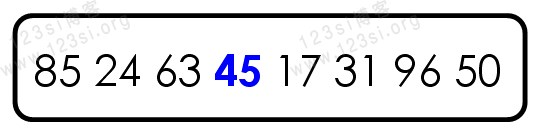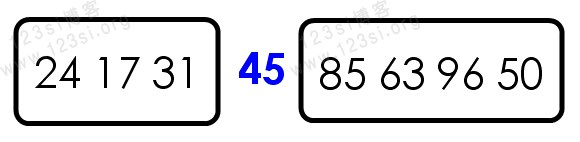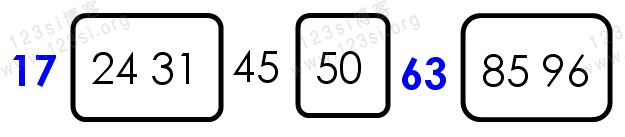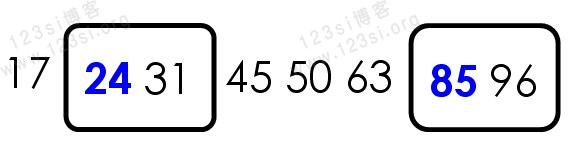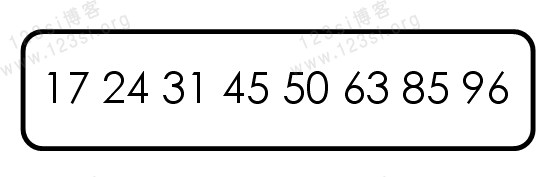#### 三、实现快速排序（Quicksort）

var quickSort = function (arr) {};

var quickSort = function (arr) {    if (arr.length <= 1) { return arr; }};

var quickSort = function (arr) {    if (arr.length <= 1) { return arr; }    var pivotIndex = Math.floor(arr.length / 2);    var pivot = arr.splice(pivotIndex, 1);    var left = [];    var right = [];};

var quickSort = function (arr) {    if (arr.length <= 1) { return arr; }    var pivotIndex = Math.floor(arr.length / 2);    var pivot = arr.splice(pivotIndex, 1);    var left = [];    var right = [];    for (var i = 0; i < arr.length; i++) {        if (arr[i] < pivot) {            left.push(arr[i]);        } else {            right.push(arr[i]);        }    }};

var quickSort = function (arr) {    if (arr.length <= 1) { return arr; }    var pivotIndex = Math.floor(arr.length / 2);    var pivot = arr.splice(pivotIndex, 1);    var left = [];    var right = [];    for (var i = 0; i < arr.length; i++) {        if (arr[i] < pivot) {            left.push(arr[i]);        } else {            right.push(arr[i]);        }    }    return quickSort(left).concat([pivot], quickSort(right));};#### 四、知识点

• JavaScript 的除法运算“/”可以返回小数。
• JavaScript Math 对象的floor()方法可对一个数进行下舍入。
• JavaScript Array 对象的concat()方法用于连接两个或多个数组。该方法不会改变现有的数组，而仅仅会返回被连接数组的一个副本。
• JavaScript Array 对象的splice()方法向/从数组中添加/删除项目，然后返回被删除的项目。该方法会改变原始数组。

#### 五、快速排序（Quicksort）完整示例

<!DOCTYPE html><html><head>    <meta charset="UTF-8">    <meta name="viewport" content="width=device-width, initial-scale=1.0">    <meta http-equiv="X-UA-Compatible" content="ie=edge">    <title>快速排序</title>    <script type="text/javascript">        var quickSort = function (arr) {            if (arr.length <= 1) { return arr; }            var pivotIndex = Math.floor(arr.length / 2);            var pivot = arr.splice(pivotIndex, 1);            var left = [];            var right = [];            for (var i = 0; i < arr.length; i++) {                if (arr[i] < pivot) {                    left.push(arr[i]);                } else {                    right.push(arr[i]);                }            }            return quickSort(left).concat([pivot], quickSort(right));        };        var arr = [85, 24, 63, 45, 17, 31, 96, 50];        console.log("将数组从小到大排序：");        console.log(arr)        console.log("快速排序（Quicksort）结果：");        console.log(quickSort(arr));    </script></head><body>    <h1>快速排序</h1></body></html>

（完）